# What is the Compound Interest Formula?

Definition:

The compound interest formula, A=P(1+r/n)^nt, lets you quickly calculate the value of your total funds, aka the principal plus interest, when the interest is compounded over that time period.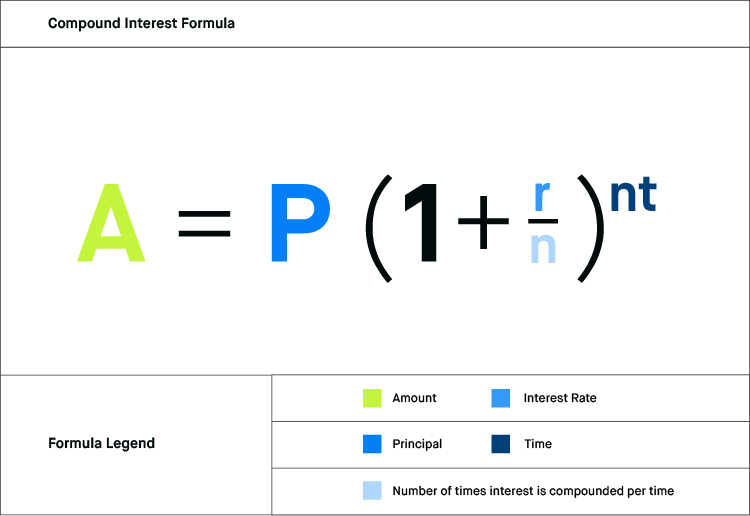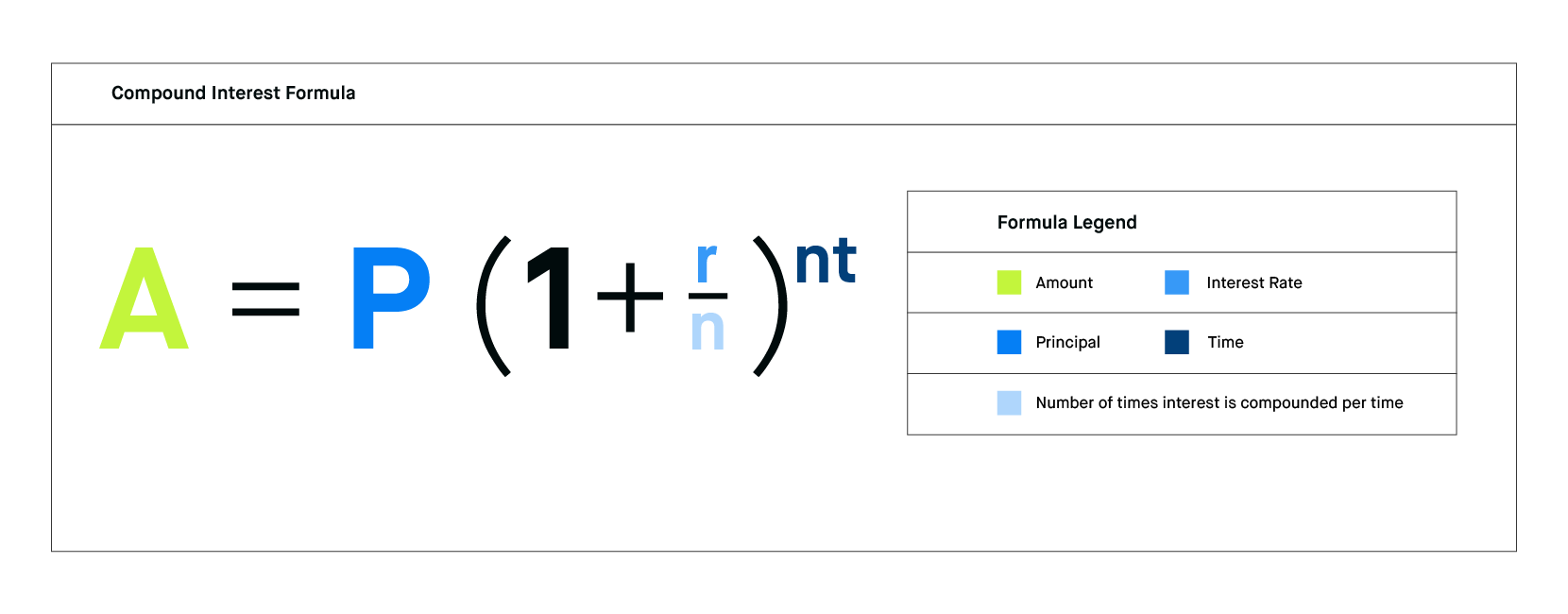## 🤔 Understanding the compound interest formula

The mathematical formula for calculating compound interest, A=P(1+r/n)^nt, uses four simple numbers to allow you to see how much money plus interest you’ll have after the number of time periods, or compound periods. ‘A’ represents the accrued amount of your principal plus interest, which is the total. ‘P’ stands for the principal, which is your original amount invested. The ‘r’ shows the interest rate in decimal form. The ‘n’ variable is used in two places and stands for the number of compounding periods. The ‘t’ represents the time in years. Together, these variables allow you to calculate your accrued amount for any amount of time and interest rate.

Example

Consider for a moment that your grandmother is a firm believer in saving money and refuses to give gift cards or cash at holidays. Instead, she gives all of the grandchildren \$500 invested in five-year CDs (Certificates of Deposit) with compound interest figured twice a year and a 2% interest rate. Set aside the delayed gratification for a moment -- The question is, how much money will the CD be worth in 5 years? That is where compound interest kicks in.

Filling in the compound interest formula of A=P(1+r/n)^nt, your holiday gift looks like this: A = 500(1+.022)^25. Following the order of operations, we calculate the amount in the parenthesis first to get A = 500(1.01)^25. From there, we input the exponents to get A = 500(1.10). Now in our final calculation, the final value is A = \$550. At the end of 5 years, your CD will be worth \$550.

## Takeaway

Compound interest is kind of like a snowball rolling quickly downhill…

A small snowball is rolled from the top of a tall mountaintop and speeds downhill, gathering more along the way. Then, that new snow picks up more snow, and the snowball that crashes at the bottom of the mountain is many times larger than the snowball rolled at the top. The compound interest formula (A=P(1+r/n)^nt) is the math that tells you how big that avalanche will be when it gets to the bottom.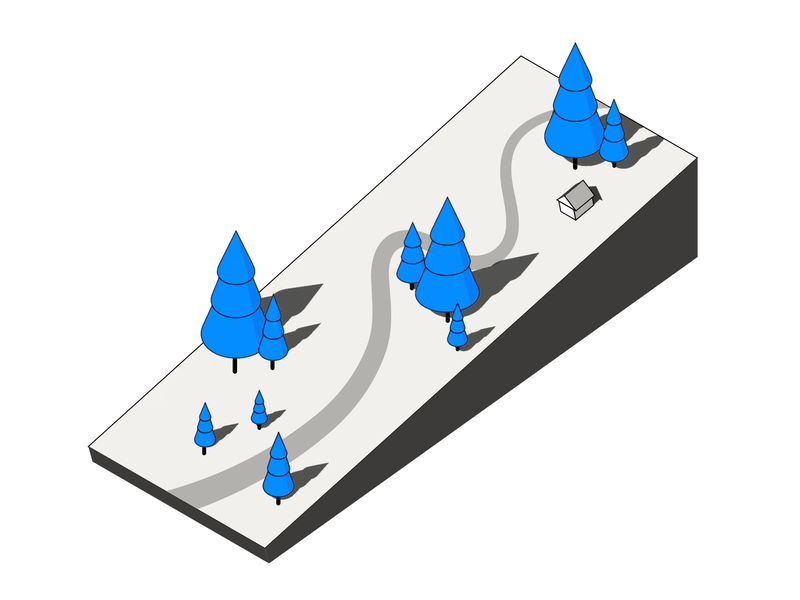Certain limitations apply

The free stock offer is available to new users only, subject to the terms and conditions at rbnhd.co/freestock. Free stock chosen randomly from the program’s inventory.

Tell me more…

## What is the difference between simple and compound interest?

Simple interest and compound interest can be gained by funds over time, based on the fund’s initial amount (whether it is as a cost to a loan or an increase in funds in an investment or savings) the interest rate, and the number of compounding periods. The two forms of interest differ, because simple interest is static, and compound interest is dynamic. With simple interest, the interest earned is removed or ignored when calculating the next interest cycle. In other words, interest is only calculated on your original principal amount. With compound interest, the previous interest earned is added back to the principal for the next interest cycle.

There is an old mental game that supposes you save a penny a day. Save one penny the first day, two pennies the second day, three pennies on the third day, and so on. That is a little like compound interest being added back to the principal (although the interest rate is not reflective of the real world and is only to illustrate the concept) if you had daily compounding periods.

For example, the second day two pennies of interest would be added to your deposited penny, the third day three pennies would be added to the total you had the day before, and so on. The interest grows because it is earned on the interest previously added plus the initial deposit. At the end of one year, that mental game results in a total of \$667.95 saved from the initial penny investment. If we use a version of this game more similar to simple interest, you save one penny the first day, one penny of interest is added the second day, one penny of interest is added on the third day, and so on. In that case, the interest never changes even as the total in the account grows. With the simple interest version, you would only have \$3.65 at the end of the year from the initial penny of savings.

## What are compounding periods?

Compounding periods are simply the length of time between the points when interest is calculated on an investment or loan. Compounding periods should be specified in the loan, savings, or other investment agreement documents. Some commonly used compounding periods are annually (once per year), semi-annually (twice a year), quarterly (four times a year), and monthly (twelve times a year).

More frequent compounding periods increase the speed at which the initial investment amount grows. In fact, compounding periods are so important to the way that compound interest works that it must be used twice in the compound interest formula to account for its effect.

Loans with more frequent compounding periods also increase the amount of interest owed. That usually means higher minimum payments are required to reduce the total owed. When taking out a loan, a lower number of compounding periods would generally be preferable as it results in less interest owed. On an investment, more compounding periods are typically sought to more quickly grow the funds.

## How do you calculate simple and compound interest?

Simple interest and compound interest have very similar formulas. The compounding effect is the difference between the two interest formulas. The simple interest formula is A = P(1 + rt) while the compound interest formula is A = P(1+r/n)^nt. The variables in both formulas represent the same concepts. ‘A’ stands for accrued amount (your principal plus the interest earned). ‘P’ represents the principal (your original amount). The ‘r’ shows the interest rate in decimal form. The small ‘t’ represents the time in years. The additional variable in the compound formula is ‘n,’ the number of compounding periods per year.

Consider that a baby inherits \$10,000 from a great aunt and her parents want to put the inheritance in savings until the baby is 18. Two banks in their neighborhood offer savings account choices. Bank A and Bank B both offer 2% interest. Bank A has simple interest, and Bank B has compound interest with quarterly compounding periods. The simple interest formula shows that if they choose Bank A with simple interest, the account will have \$13,600 at the end of 18 years. \$13,600 = \$10,000(1+(.02x18)). The compound interest formula shows that if they choose Bank B with compound interest, the account will have \$14,320.44 at the end of 18 years. \$14,320.44 = \$10,000(1+(.02/4))^(4x18).

## How do you calculate compound interest using Excel?

Excel makes it easy to create a compound interest calculator without any programming skills. To figure compound interest in Excel, you will need a cell for each variable and a cell for the answer (formula). Because of the way Excel functions, there is a specific process that must be used rather than just pasting the formula and assigning cells.

To begin, open a new spreadsheet. In cell A1, type “Compound Interest Calculator” as a label for the sheet. This is useful if you later create new sheets with simple interest or other interest formulas such as continuous compound interest. Next, enter labels for each variable and one for total in column A. Principal (A3), rate (A4), time (A5), number of compounding periods per year (A6), and total (A8) should each have specific cells as indicated in the parentheses.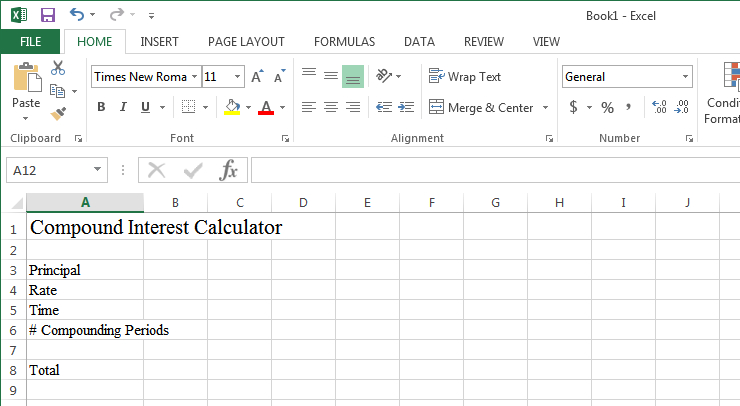To enter the formula, click on cell B8 (besides the total label). In the data entry bar, click the fx button and type future value in the formula search box. Select ‘FV’ and ‘go,’ and a pop-up box showing several variable options will open.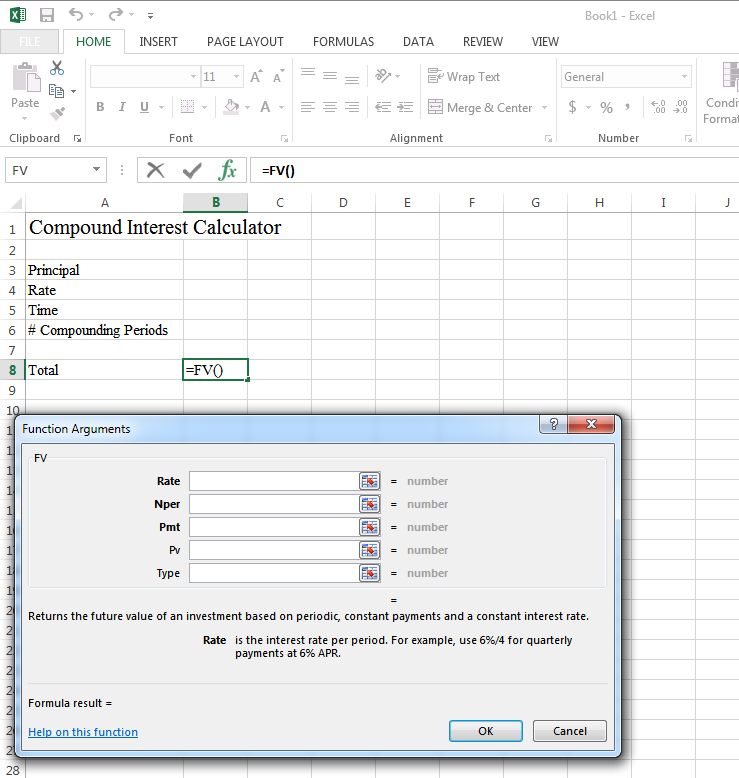In the ‘rate’ section click cell B4 type " / "and then click cell B6. That section tells Excel the r/n portion of the formula. In the ‘nper’ section click cell B5, type " * "and then click cell B6. The ‘nper’ section tells Excel the exponent, nt, part of the formula. Leave the ‘pmt’ field blank. In the ‘pv’ (present value) field, click cell B3. Leave the ‘type’ field blank and click Ok. Until you enter values, the formula cell will show an error.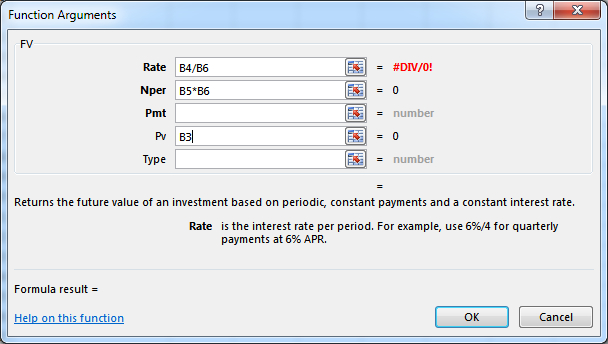Now that the formula is complete you’ll need to enter your variables. For the principal amount, you must enter this number as a negative. This is part of the Excel coding and nothing that can be changed. Think of it as the money left your pocket, so it's negative for the sake of the formula. Enter the interest rate in decimal form instead of as a percentage or whole number. Be sure to format your variable and total cells to your preference. You can format a cell by right-clicking on it and then choosing the format. From there, you can adjust the currency formatting, red for negative option, the number of decimal places/rounding, and more.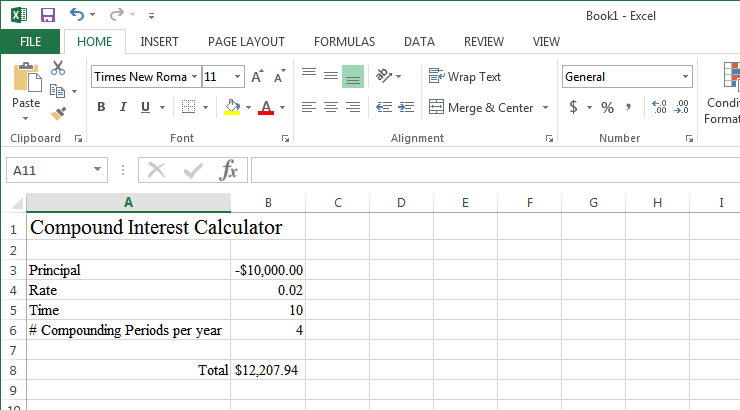## What’s the formula for continuous compound interest?

Continuous compound interest is a theoretical practice used as one way to help compare possible investment growth at different time and rate options. The formula for continuous compound interest is P = e^rt. The e stands for a mathematical constant and is often written out to only four decimal places, 2.7183, much like Pi is only written to two decimal places. The ‘e’ will not change in this formula, regardless of the other values used. ‘P’ stands for principal, ‘r’ stands for the interest rate in decimal form, and ‘t’ stands for the amount of time in years.

The continuous compound interest model can approximate the action of continual reinvestment rather than true compound interest earned on a set schedule of compounding periods. Sometimes it’s used as a shortcut to roughly approximate investment growth. For example, when the number of compounding cycles is unknown.

## What is the “Rule of 72”?

The Rule of 72 is a financial rule of thumb to roughly estimate how many years are required to pass for the value of an investment to double. The rule relies on fixed interest rates, and the result is not entirely accurate. The Rule of 72 works by dividing 72 by the annual rate of return (as a whole number). For example, the Rule of 72 says it will take 14.4 years for an investment at 5% return to double (72/5). The exact time could vary based on compounding frequency. The Rule of 72 becomes less accurate as the rate of return rises with returns over 20% becoming unfavorable for the method.

Like all rule of thumb calculations, there are variations of the method. For compound interest investments, the rule of 69.3 is considered more accurate by some investors. The Rule of 69.3 works just like the Rule of 72 but uses 69.3 as the starting number instead of 72. For example, the Rule of 69.3 says it will take 13.38 years for an investment at 5% return to double (69.3/5). Again, compounding frequency affects accuracy.

Keep in mind that these rules are shown for illustrative purposes only and they don’t guarantee that any investment will double.

## What is the compound annual growth rate (CAGR)?

Sometimes investors want to know what rate of return is needed to reach a particular value at a specific time. For example, if saving for retirement, a couple might want to know what rate of return would be needed to reach their savings goal before their planned retirement year. The compound annual growth rate (CAGR) formula figures the required rate of return to reach a goal. The compound annual growth rate formula tells the rate of return necessary to move from the principal amount (the beginning balance) to the desired accrued amount (the ending balance) in a specific amount of time.

The formula to calculate compound annual growth rate (CAGR) is:

CAGR = (EB/BB)^(1/n)-1

‘EB’ represents the ending balance, and ‘BB’ stands for the beginning balance. The ‘n’ represents the desired time in years. For example, if a couple wanted to invest \$250,000 towards retirement and hope to have \$1 million for retirement in 20 years, they would need a 7.18% CAGR to meet that goal.

7.18% = (1,000,000/250,000)^(1/20) -1

CAGR is limited because it assumes a continuously stable growth rate, and many investments do not have a guaranteed return or steady return rate. Instead, many investments fluctuate in rate of return. However, CAGR can be useful in comparing the overall performance of two different investments over time. CAGR can also reveal problems with investing goals if the rate of return needed is far outside average returns in a specific type of investment. For example, if the couple above hypothetically wanted to meet their goal through only investing in an investment a friend told them had returns of 5%, it is unlikely they would meet their goal. However, as with any investment, past performance is not indicative of future results.

## What is annual percentage yield (APY)?

Annual percentage yield (APY) is a way of expressing the amount earned on a compound interest-bearing investment in a year. APY takes the base interest rate (APR - annual percentage rate) and makes adjustments for the effect of compounding and the compounding frequency on the total. The result is a percentage rate that is higher than the raw APR.

Formulas such as the compound interest formula rely on the base interest rate, the APR, not the annual percentage yield. Using the APY in formulas asking for the raw interest rate or APR can lead to incorrect results. Care should always be taken to ensure if APR or APY is being discussed when investing.

In financial advertising, loans and credit card rates are often show as APR. However, the APY can be drastically higher based on compounding frequency. Some credit cards compound debt daily, quickly inflating the APY. For investments and savings, advertisements often feature APY to showcase the result of the investment in a more positive light. Both methods are accurate but require the customer to understand the difference in APR and APY.

## Do stocks earn compound interest?

While some stocks do pay dividends, stocks do not earn any interest, either simple or compound. Stocks are an investment without any return guarantee and may even decrease in value in some conditions.

The only way to earn “income’ on stocks is to invest in stocks that pay out dividends, which could be reinvested by purchasing more stock. There are different classes of shares of stocks. Preferred stock shareholders typically get first dibs on dividends, but even common stock can be eligible to receive dividends in some cases.

Some brokers offer customers the ability to set up automatic reinvestment of dividends. This is often done with something called a dividend reinvestment plan (DRIP). By reinvesting dividends, those funds can begin to earn income in a pattern that’s similar to the way compounded interest. However, unlike an interest-bearing account, there are no guarantees that value will increase, and there is a chance of value loss on both the original investment and newly reinvested dividends.

## What are some compound interest calculator apps?

Understanding the compound interest formula and all of its uses can be a helpful tool in understanding investing options. Sometimes the actual calculations are just more straightforward with a calculator. There are numerous financial calculator apps available for both Apple and Android devices. No one app is perfect, but there are some useful free apps available. Keep in mind that some of the free apps do contain ads.

Also, you can find a compound interest calculator on the Securities and Exchange Commission’s Investor.gov site: https://www.investor.gov/additional-resources/free-financial-planning-tools/compound-interest-calculator

The above examples are for illustrative purposes only and do not reflect the performance of any investment. Investing involves risk - aka you could lose your money.

Please be advised that by clicking a third-party URL or hyperlink, you’ll be accessing a third-party website. Robinhood Financial LLC is not implying that any monitoring is being done by Robinhood Financial LLC of any information contained on the third-party website. Robinhood Financial LLC is not responsible for the information contained on the third-party website or your use of or inability to use such site. Nor do we guarantee their accuracy and completeness. 20191129-1023955-3085467

The free stock offer is available to new users only, subject to the terms and conditions at rbnhd.co/freestock. Free stock chosen randomly from the program’s inventory.

## Related Articles

What is a Dividend?
Updated September 28, 2020

## You May Also Like

The 3-minute newsletter with fresh takes on the financial news you need to start your day.
The 3-minute newsletter with fresh takes on the financial news you need to start your day.

© 2020 Robinhood Markets, Inc. Robinhood® is a trademark of Robinhood Markets, Inc.

This information is educational, and is not an offer to sell or a solicitation of an offer to buy any security. This information is not a recommendation to buy, hold, or sell an investment or financial product, or take any action. This information is neither individualized nor a research report, and must not serve as the basis for any investment decision. All investments involve risk, including the possible loss of capital. Past performance does not guarantee future results or returns. Before making decisions with legal, tax, or accounting effects, you should consult appropriate professionals. Information is from sources deemed reliable on the date of publication, but Robinhood does not guarantee its accuracy.

Robinhood Financial LLC provides brokerage services. Robinhood Securities, LLC, provides brokerage clearing services. Robinhood Crypto, LLC provides crypto currency trading. All are subsidiaries of Robinhood Markets, Inc. ('Robinhood').

1200251